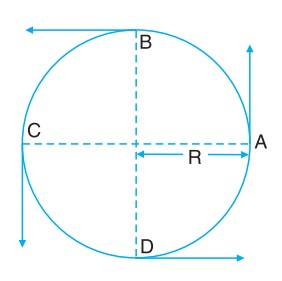# Uniform Circular Motion

Consider the motion of an object along a circular track of radius R through four points A, B, C and D on the track. If object completes each round of motion in same time, then it covers equal distance in equal interval of time and its motion will be uniform motion.

Since during this uniform motion equal distance is being covered in equal interval of time, therefore, the ratio of distance covered to the time taken i.e., speed will remain constant. It means in uniform circular motion speed remains constant.Velocity remains along the direction of motion. The direction of motion changes at every point A, B, C and D. Since there is a change in direction of motion, therefore, the direction of velocity also changes.

In uniform circular motion, velocity changes due to change in direction of motion and the motion of the object is accelerated motion. This acceleration is due to change in the direction of motion. But in this motion speed remains constant.